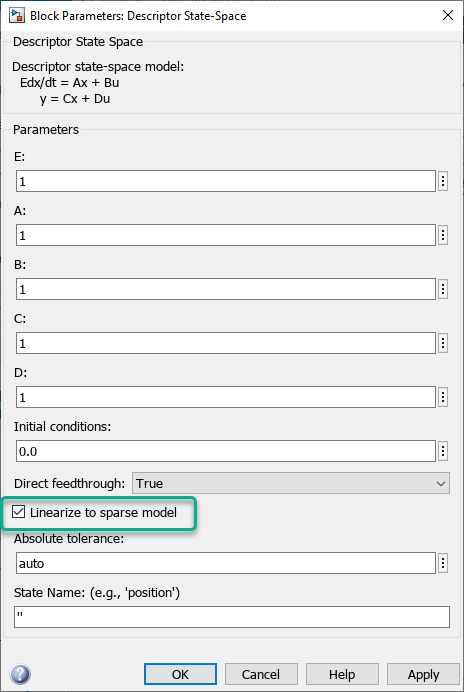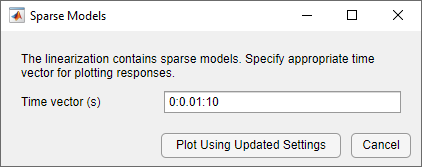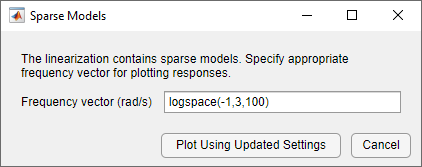## Linearize Sparse Models

You can obtain a sparse linear model from a Simulink® model that contains a Sparse Second Order or Descriptor State-Space block. Linearizing such models produces one of the following sparse state-space objects.

For a command-line linearization example, see Linearize Simulink Model to a Sparse Second-Order Model Object.

The Sparse Second Order block always linearizes to a `mechss` model. As a result, the overall linearized model is a second-order sparse model when this block is present.

To linearize a Descriptor State-Space block to a sparse model, select the Linearize to sparse model block parameter.You can also select this parameter programmatically. Here, `blockpath` is the path to the Descriptor State-Space block.

`set_param(blockpath,'LinearizeToSparse','on')`

The Linearize to sparse model parameter of the Descriptor State-Space block is ignored when you specify a custom linearization for the block.

### Linearize Sparse Models at the Command Line

You can linearize a model that contains a sparse block at the command line using either the `linearize` function or an `slLinearizer` interface.

To analyze the resulting linearized model in the:

• Time domain, you must specify a time vector or a final simulation time. For example, plot the step response of linearized sparse model `linsys` for 10 seconds using 100 sample points.

```t = linspace(0,10,1000); step(sys,t)```
• Frequency domain, you must specify a frequency vector. For example, plot the Bode response of linearized sparse model `linsys` from 101 to 105 rad/sec using 1000 logarithmically spaced frequency points.

```w = logspace(1,5,1000); bode(linsys,w)```

For more information on analyzing sparse models at the command line, see Sparse Model Basics.

### Linearize Sparse Models Using Model Linearizer

You can interactively linearize a model that contains a sparse block using the Model Linearizer app.

Each time you plot a time-domain or frequency-domain response of the resulting linearized model, Model Linearizer prompts you to enter a time or frequency vector for plotting. For example:

• Time domain — Plot response for 10 seconds in 0.01 second increments.• Frequency domain — Plot response from 10–1 to 103 rad/sec using 100 logarithmically spaced frequency points.You cannot change the time or frequency vector for an existing sparse model response plot. Instead, create a new plot for the same sparse model using an updated time or frequency vector.

To view the structure of the linearized sparse model, export the model to the MATLAB® workspace and use the `spy` and `showStateInfo` functions.

### Limitations

Sparse linearization has the following limitations.

• If you disable block reduction when linearizing a sparse model, the resulting linear model is a dense `ss` model object.

• Due to simulation limitations, snapshot linearization might not work when your model contains a Descriptor State-Space or Sparse Second Order block.

• Sparse linearization is incompatible with block substitutions involving tunable or uncertain models, such as `genss` or `uss` (Robust Control Toolbox), respectively.

• When analyzing linearized sparse systems, Pole-Zero Map and I/O Pole-Zero Map plots are not supported.

## Related Topics